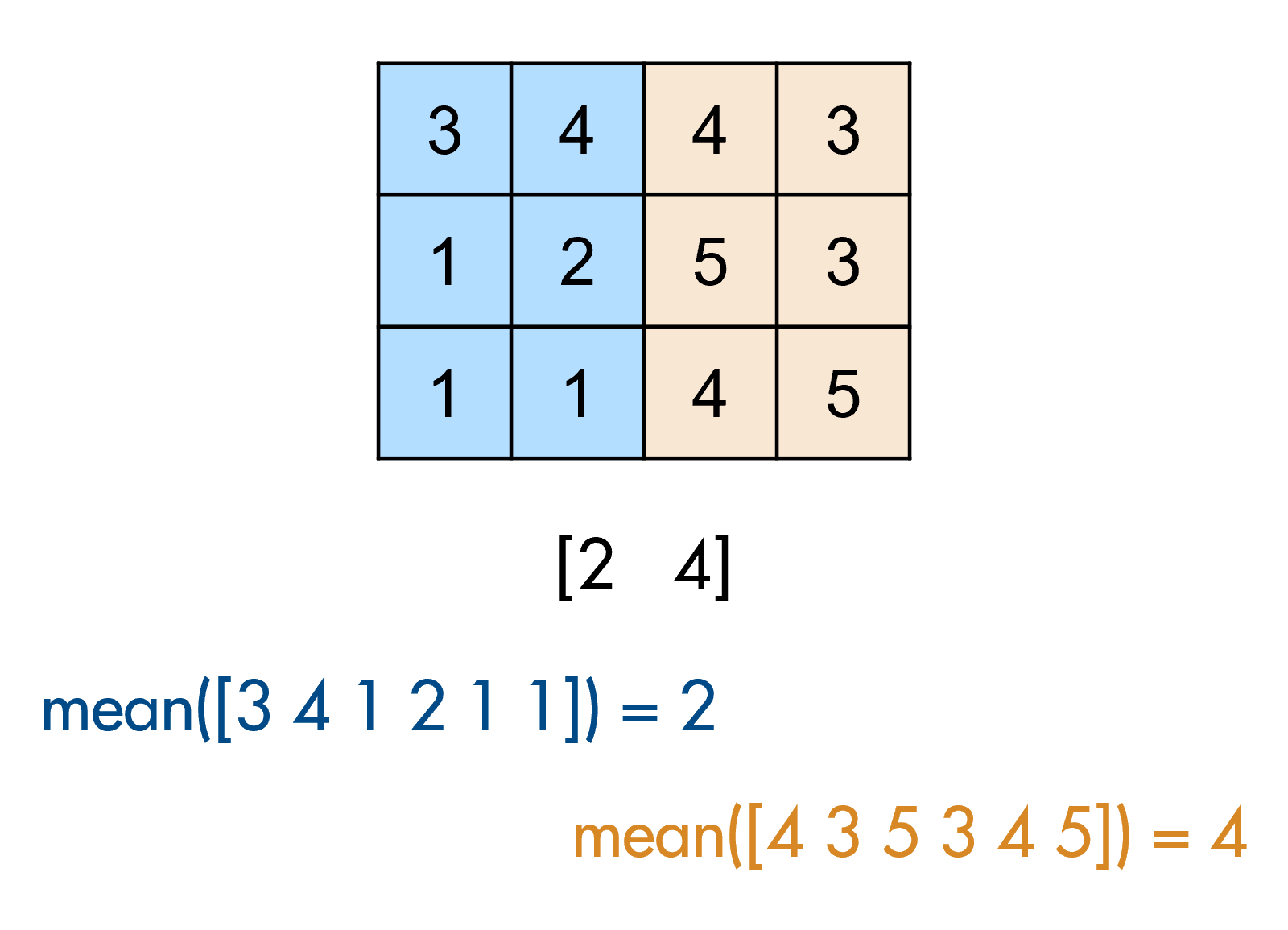# Problem 55240. Calculate the mean of each half of a matrix

Given a matrix with an even number of columns, n, return a 1-by-2 row vector where the first element is the mean of all the elements in the first n/2 columns, and the second element is the mean of all the elements in the second n/2 columns.
For exampleA = [3 4 4 3;
1 2 5 3;
1 1 4 5];
m = halfmeans(A)
m =
2 4
(2 = mean of [3,4,1,2,1,1], 4 = mean of [4,3,5,3,4,5])

### Solution Stats

51.49% Correct | 48.51% Incorrect
Last Solution submitted on Dec 01, 2023

### Community Treasure Hunt

Find the treasures in MATLAB Central and discover how the community can help you!

Start Hunting!